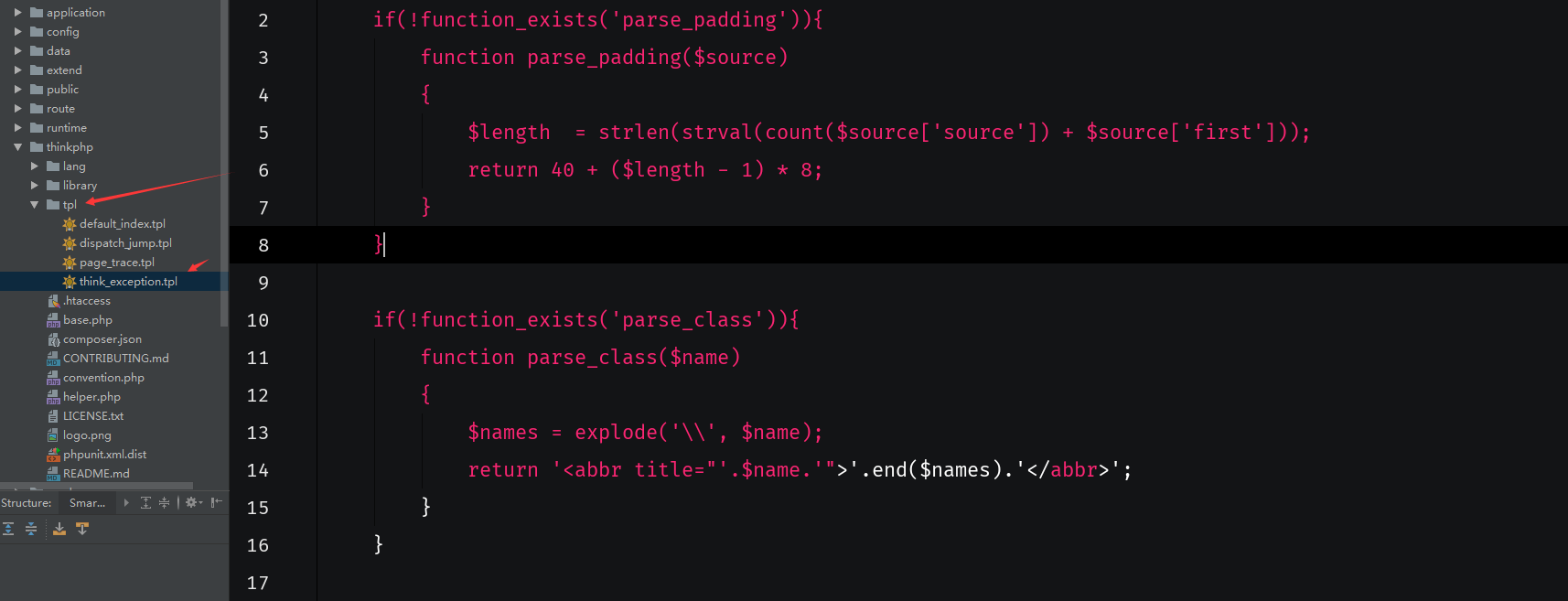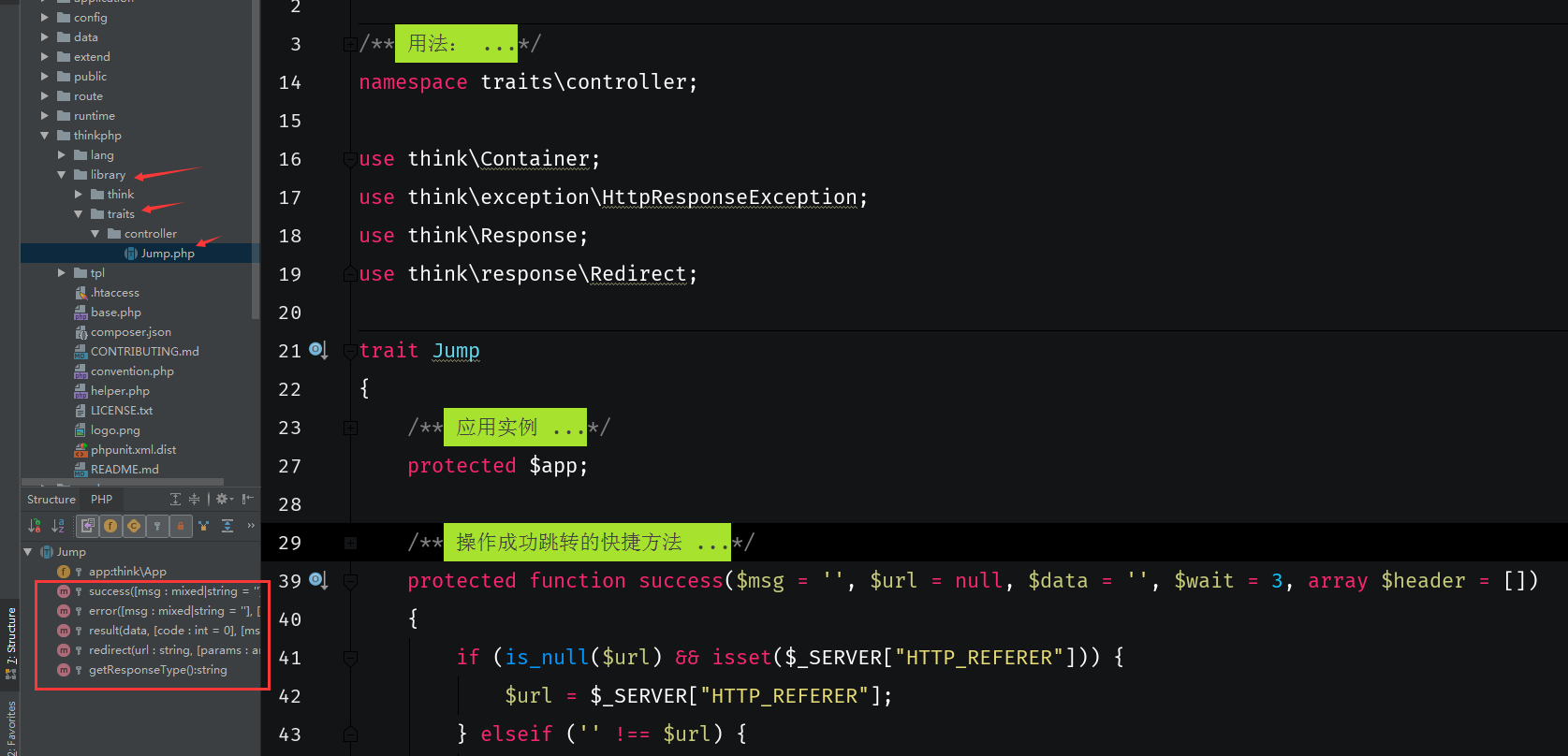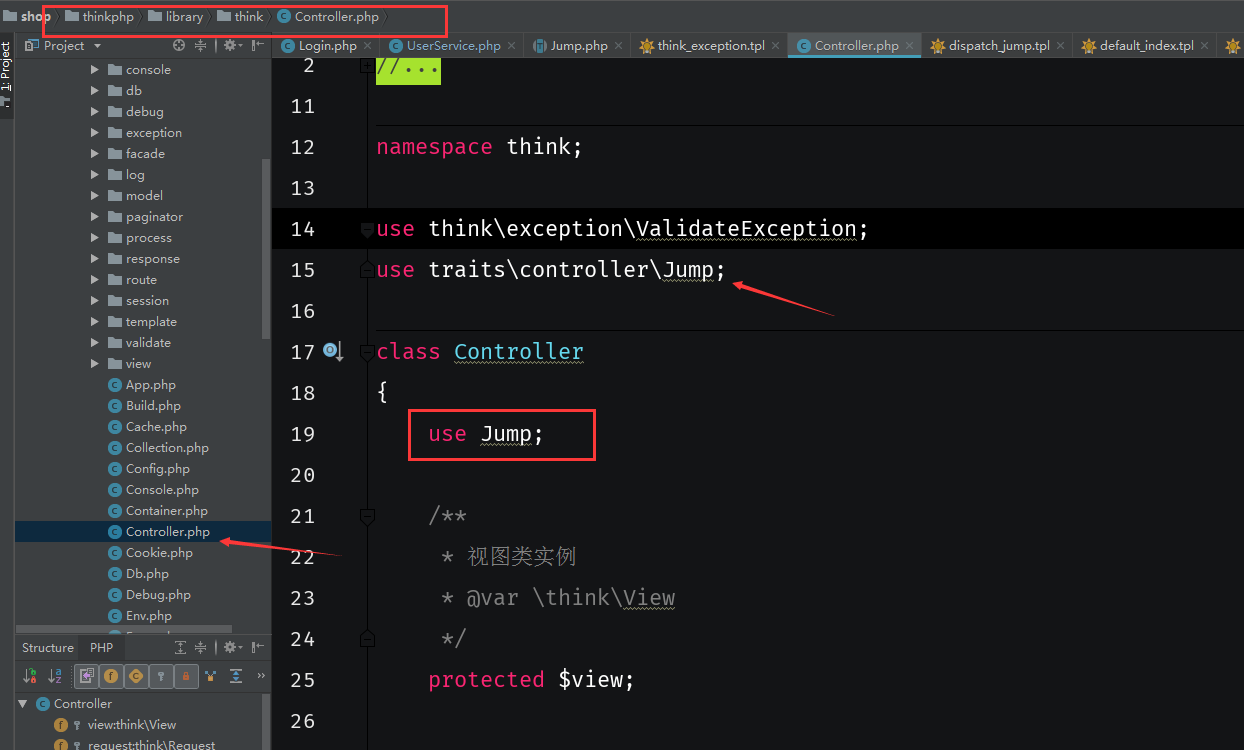超类：

超类就是定义了一些通用的方法，在其他类中不需要继承就可以直接使用的超类的方法，而是通过use引入的类，相当于include这样理解

$this->success($msg,$url);$this->error($msg,$url);

$this->result($data,$code,$msg,$type);$this->redirect($url,$param);/**
* 用法：
* class index
* {
*     use \traits\controller\Jump;
*     public function index(){
*         $this->error(); *$this->redirect();
*     }
* }
*/